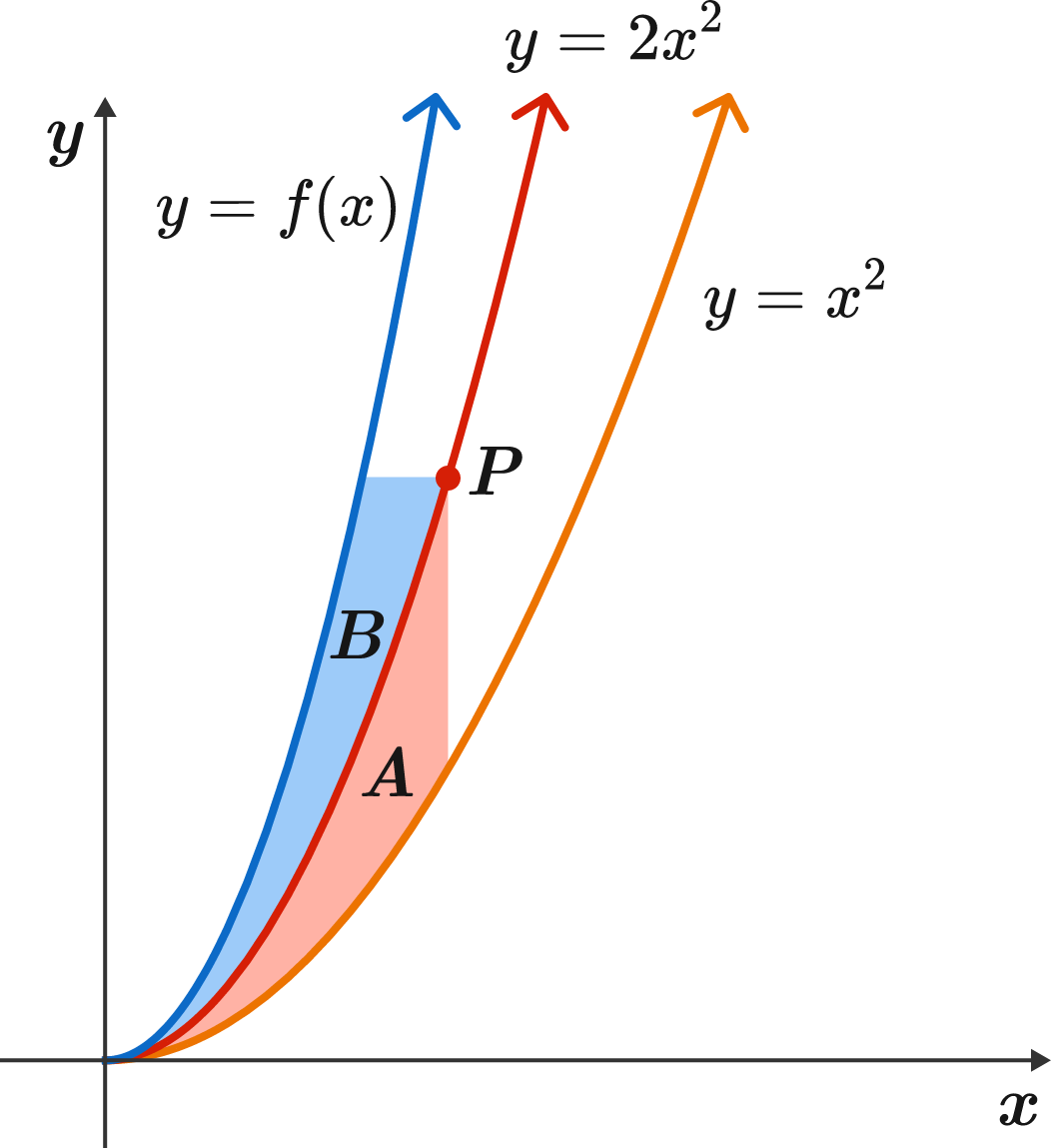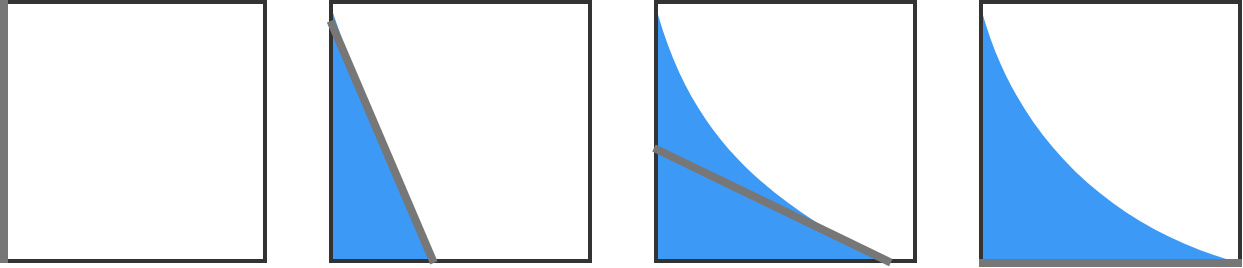# Problems of the Week

Contribute a problemA monkey is typing a string of random letters, with all letters equally (and independently) likely to be typed.

Which word is more likely to appear first in the string, heart or earth?

Take a point $P$ on the graph of $y=2x^2,$ and draw a line going through $P$ parallel to the $y$-axis. Then call the red area $A:$ the area bounded by this line, the graph of $y=x^2,$ and the graph of $y=2x^2.$

Now, draw a line going through $P$ parallel to the $x$-axis. Then call the blue area $B:$ the area bounded by this line, the graph of $y=2x^2,$ and the graph of $y=f(x).$

This function $f(x)$ is continuous and displays the unique property that for every point $P$ on $y = 2x^2,$ the two areas $A$ and $B$ are equal.

Find $f(x)$ and evaluate $f(12).$An infinitely thin, $k\text{ cm}$-long squeegee begins to slide down from the upper-left corner of a $k\text{ cm} \times k\text{ cm}$ square window. Its other end simultaneously slides toward the lower-right corner of the window, with the upper end kept in contact with the left side of the window.

If $k=\sqrt{\dfrac{2016}{\pi }}$, what is the area of the window $\big($in $\text{cm}^2\big)$ cleaned by the squeegee?Note: you may end up with an integral expression that's difficult to evaluate analytically. Feel free to finish the job using the code environment below:

import math
Python 3
You need to be connected to run code

Evaluate the following integral:

$\large \int_{ - \infty}^{\infty} \frac{\cos x}{1+x^2} dx.$

In the country of Brilliantia, there are $n > 60$ airports built recently such that the distances between any two airports are all different from one another.

A plane will always fly from an airport to the nearest airport. Let an origin of an airport $\text{A}$ be another airport that flies planes to $\text{A}$.

What is the maximum number of origins an airport can have?

×